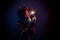Photo by Matt Palme

# The Wizardry of Elliptic Curve Cryptography

The flexibility of Elliptic Curve Cryptography (ECC) just seems endless. In places, it almost feels like wizardry. So let’s look at the wizardry involved in ElGamal encryption matched to ECC.

First Alice creates her key pair (a and aP), and where a (her private key) is a scalar, and P is a point on the elliptic curve.

`a := suite.Scalar().Pick(suite.RandomStream()) A := suite.Point().Mul(a, nil)`

Her pubic key is the point P, added to itself a times. She passes her public key to Bob (aP). He first takes the message and converts it into a byte array, and matches it to an elliptic curve point:

`M := group.Point().Embed(message, random.New())`

And then, as if by magic, he uses some randomization and creates a new elliptic curve point (k), and creates two cipher element (K and C):

`k := group.Scalar().Pick(random.New()) K= group.Point().Mul(k, nil)          S := group.Point().Mul(k, pubkey)     C = S.Add(S, M)`

Bob then pass that back to Alice. She uses her private key (a) to decipher the message and pluck it back off the elliptic curve (with the Data() method):

`S := group.Point().Mul(prikey, K) M := group.Point().Sub(C, S)      message, err = M.Data()`

It is beauty in the form of maths. A demo of the method is here:

## The detail

With ECC, we take points on a defined curve — such as Curve 25519 — and then perform point addition and subtraction. So how can we convert the ElGamal method into ECC? First, Alice first creates a private key (a) — and which is a random scalar value — and a public key (aP) — and which is a point on the elliptic curve. PP is the base point on a curve. Alice’s public key will then be:

A=aP

If Bob wants to send Alice an encrypted message (M), he creates a random value (k) and uses her public key (A) to produce a cipher messages of:

K=kP

and:

C=kA+M

and where MM is matched to a point on the elliptic curve. Now Alice receives (K and CC, and computes the shared secret (S) using her private key (a):

S=aK

and then computes the message with:

M=C−S

As C and S will be points on the elliptic curve, this will be done with a point subtraction operation. Overall this will recover the original message as:

C−S=kA+M−aK=kaP+M−akP=M

The following is the code [taken from here]:

`package mainimport (    "fmt"    "os"    "go.dedis.ch/kyber"    "go.dedis.ch/kyber/group/edwards25519"    "go.dedis.ch/kyber/util/random")func ElEncrypt(group kyber.Group, pubkey kyber.Point, message []byte) (    K, C kyber.Point, remainder []byte) {    // Embed the message (or as much of it as will fit) into a curve point.    M := group.Point().Embed(message, random.New())    fmt.Printf("Message point:\t%s\n" , M.String())    max := group.Point().EmbedLen()    if max > len(message) {        max = len(message)    }    remainder = message[max:]    // ElGamal-encrypt the point to produce ciphertext (K,C).    k := group.Scalar().Pick(random.New()) // ephemeral private key    K = group.Point().Mul(k, nil)          // ephemeral DH public key    S := group.Point().Mul(k, pubkey)      // ephemeral DH shared secret    C = S.Add(S, M)                        // message blinded with secret    return}func ElDencrypt(group kyber.Group, prikey kyber.Scalar, K, C kyber.Point) (    message []byte, err error) {    // ElGamal-decrypt the ciphertext (K,C) to reproduce the message.    S := group.Point().Mul(prikey, K) // regenerate shared secret    M := group.Point().Sub(C, S)      // use to un-blind the message    message, err = M.Data()           // extract the embedded data    return}func main() {    M:="Testing"    argCount := len(os.Args[1:])    if (argCount>0) {M= string(os.Args)}        suite := edwards25519.NewBlakeSHA256Ed25519()    // Alice's key pair (a,A)    a := suite.Scalar().Pick(suite.RandomStream())     A := suite.Point().Mul(a, nil)                     fmt.Printf("Private key (Alice):\t%s\n" ,a.String())    fmt.Printf("Public key (Alice):\t%s\n" , A.String())    fmt.Printf("\n\n--- Now Bob will cipher the message for Alice\n")    fmt.Printf("Bob's message:\t%s\n",M)    m := []byte(M)    K, C, _ := ElEncrypt(suite, A, m)    fmt.Printf("\nBob cipher (K):\t%s\n" , K.String())    fmt.Printf("Bob cipher (C):\t%s\n" , C.String())    fmt.Printf("\n\n--- Now Alice will decipher the ciphertext (K,C) with her private key (a)\n")    M_decrypted, err := ElDencrypt(suite, a, K, C)    if err != nil {        fmt.Println("Error: " + err.Error())    }    fmt.Printf("\nOutput:\t%s",string(M_decrypted))}`

A sample run is:

`Private key (Alice):    7182fa86214b1672f36113d139b2f84ca6acbbf1dbe2202e2ad99665e4fdac00Public key (Alice): 31dfde321f1f56228feeacaeff9550c3d489ee5fd3e4e9d2e48fd41cfd9f09f6--- Now Bob will cipher the message for AliceBob's message:  Testing 123Message point:  0b54657374696e6720313233aca5a2888970256a3bb93cde2898f95fcdfd96efBob cipher (K): c794b9c278298dc3abd64b0e3af62a2f7390c6c51c13a491930dea6b2dbc6ce4Bob cipher (C): 27ac77843effff5b723abe990e7175ba0c7659da0f5aec98421f0a89b78f2d82--- Now Alice will decipher the ciphertext (K,C) with her private key (a)Output: Testing 123`

## ASecuritySite: When Bob Met Alice

This publication brings together interesting articles…

Written by

## Prof Bill Buchanan OBE

Professor of Cryptography. Serial innovator. Believer in fairness, justice & freedom. EU Citizen. Auld Reekie native. Old World Breaker. New World Creator.

## ASecuritySite: When Bob Met Alice

This publication brings together interesting articles related to cyber security.

Written by

## Prof Bill Buchanan OBE

Professor of Cryptography. Serial innovator. Believer in fairness, justice & freedom. EU Citizen. Auld Reekie native. Old World Breaker. New World Creator.## ASecuritySite: When Bob Met Alice

This publication brings together interesting articles related to cyber security.

## More From Medium

Medium is an open platform where 170 million readers come to find insightful and dynamic thinking. Here, expert and undiscovered voices alike dive into the heart of any topic and bring new ideas to the surface. Learn more

Follow the writers, publications, and topics that matter to you, and you’ll see them on your homepage and in your inbox. Explore

If you have a story to tell, knowledge to share, or a perspective to offer — welcome home. It’s easy and free to post your thinking on any topic. Write on Medium